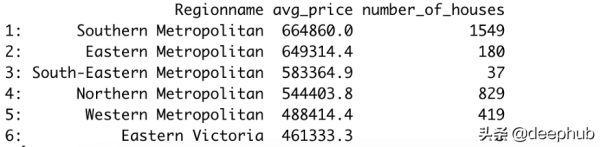# 如何比较Python中的Pandas和data.table

`# pandas  import pandas as pd  melb = pd.read_csv("/content/melb_data.csv")   # data.table  library(data.table)  melb <- fread("datasets/melb_data.csv")`

#### 示例1

`# pandas  melb["Price_per_area"] = melb["Price"] / melb["Landsize"]   # data.table  melb[, Price_per_area := Price / Landsize]`#### 示例2

`# pandas  subset = melb[(melb.Price > 1000000) & (melb.Type == "h")]   # data.table  subset <- melb[Price > 1000000 & Type == "h"]`

#### 示例3

`# pandas  melb[melb.Type == "u"].groupby("Regionname").agg(  avg_price = ("Price", "mean")  )   # data.table  melb[Type == "u", .(avg_price = mean(Price)), by="Regionname"]`pandas使用groupby函数执行这些操作。对于data.table，此操作相对简单一些，因为我们只需要使用by参数即可。

#### 示例4

`# pandas  melb[melb.Type == "u"].groupby("Regionname").agg(  avg_price = ("Price", "mean"),  number_of_houses = ("Price", "count")  ).sort_values(by="avg_price", ascending=False)   # data.table  > melb[  Type == "u",  .(avg_price = mean(Price), number_of_houses=.N),  by="Regionname"  ][order(-avg_price)]`#### 示例5

`# pandas  melb.rename(columns={"Type": "HouseType",  "Distance": "DistanceCBD"},  inplace=True)   # data.table  setnames(melb,  c("Type", "Distance"),  c("HouseType", "DistanceCBD"))`